## Recommended Posts

For this challenge, I've decided to go with the radial engines.The challenge is to solve for a single vector valued function in two dimensions that describes the paths of the pistons for a radial engine with $n$ pistons, rod lengths $L$, a hub radius $r$, and a crank radius $R$ as seen in the following image: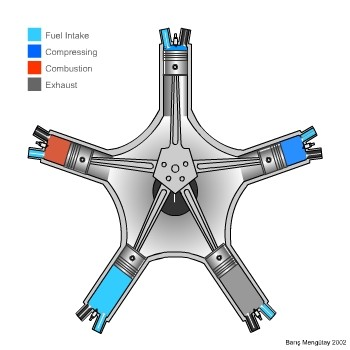All of other rods connect to the master rod, which connects to the crank. The mathematics for the path of the piston connected to the master rod is no different than a standard crank system. However, because all the other pistons are connected to rods that are attached to the master rod, the other piston paths vary slightly. Nonetheless, there is a single equation that governs the piston path for the master rod as well as the piston paths for the other rods.

I tried to find the equation using Google, but I just couldn't find it. Perhaps I wasn't looking hard enough but, if you take the time to actually figure out the vector valued equations yourself, I think you'll enjoy this challenge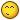Hint: The connection points on the hub for the other rods are rotated by the master rod as the crank rotates. I've also rendered a .gif using Mathematica that will help you visualize the process:Edited by Daedalus

##### Share on other sites

Why do you say there is a single equation when the vectors applied by the conrods appear to be different for the master and non master pistons?

##### Share on other sites

Why do you say there is a single equation when the vectors applied by the conrods appear to be different for the master and non master pistons?

I know it seems unlikely, but if you study the last image I posted, the one I rendered from Mathematica, then you should see how everything is related and how you can generate a single equation to govern all the piston paths. After all, I generated that animation using that single equationEdited by Daedalus

##### Share on other sites

I know it seems unlikely, but if you study the last image I posted, the one I rendered from Mathematica, then you should see how everything is related and how you can generate a single equation to govern all the piston paths. After all, I generated that animation using that single equationI can see there could well be a single equation that enables you to draw the animation, but what I notice and you allude to is that the piston paths are different, and that seems to be due to the main conrod bearings not being in identical positions with respect to the piston they are attached to.

In the animation there are no forces of friction or inertial forces to overcome. I think if you factor these into the animation you will see that the forces transmitted through the conrods is not the same in all positions.

##### Share on other sites

I can see there could well be a single equation that enables you to draw the animation, but what I notice and you allude to is that the piston paths are different, and that seems to be due to the main conrod bearings not being in identical positions with respect to the piston they are attached to.

In the animation there are no forces of friction or inertial forces to overcome. I think if you factor these into the animation you will see that the forces transmitted through the conrods is not the same in all positions.

In this challenge, I'm not asking you to apply forces or to consider tolerances within an actual engine but, just because the force on each piston is unequal, doesn't mean that the equation is wrong. Since all of the parts are connected, when one part moves the others must move to the position defined by the equation. If they didn't, then rods would get broken or pistons would have the rod separated from them or worse. Again, we are considering that each part is rigid and has no give between connection points. So, when the crank is at a given angle, all of the pistons must be located exactly where the equation specifies. After all, the challenge is only considering the number of pistons $n$, the length of the rods $L$, the radius of the hub $r$, and the radius of the crank $R$. There is no need to complicate it.

Edited by Daedalus

##### Share on other sites

It is a fascinating challenge Daedalus - still mulling it over. It is too complex for me but I am enjoying worrying away at the smaller bits I can get my teeth into.

BTW2 - Did you work out equations for both the forms above? I presume you noticed that the top animated gif does not have a master cylinder - all the connexions are hinged; whereas in the blow,suck,squeeze,bang diagram and your mathematica gif there is a fixed master cylinder. I wonder if the difference is important. Again - please don't give the answer to even this bit away.

##### Share on other sites

It is a fascinating challenge Daedalus - still mulling it over. It is too complex for me but I am enjoying worrying away at the smaller bits I can get my teeth into.

BTW2 - Did you work out equations for both the forms above? I presume you noticed that the top animated gif does not have a master cylinder - all the connexions are hinged; whereas in the blow,suck,squeeze,bang diagram and your mathematica gif there is a fixed master cylinder. I wonder if the difference is important. Again - please don't give the answer to even this bit away.

I won't give out the answer now that I know you're working on itAs for having a master rod or not, yes, I did work out both equations. The bonus for this challenge is to work out the equation when there isn't a master rod. e.g. all the rods connect to a free floating hub.

I'll give you a hint:

As you know, the piston paths are constrained to lines that intersect at the origin (the center of the shaft) where the angle of each line is defined by the number of pistons the radial engine has $\theta = i\,\frac{2\,\pi}{n}$ where $i$ is the zero based index of the piston and $n$ is the number of pistons. To simplify the math, I rotated the coordinate system by projecting the vector equations onto the following vectors, which transforms the coordinate system such that each path is along the $X$ axis:

$\vec{X}=\text{cos}\left(i\,\frac{2\,\pi}{n}\right)\,\widehat{x}+\text{sin}\left(i\,\frac{2\,\pi}{n}\right)\,\widehat{y}$

$\vec{Y}=-\text{sin}\left(i\,\frac{2\,\pi}{n}\right)\,\widehat{x}+\text{cos}\left(i\,\frac{2\,\pi}{n}\right)\,\widehat{y}$

You should notice that the vectors that I am using for the new axes have a magnitude of 1. This simplifies the vector projection operation to performing a dot product without having to consider magnitude.As for the bonus challenge with the free floating hub, I am not considering friction or forces generated by the pistons that would cause the hub's angle to change. Here's the .gif I rendered in Mathematica for the bonus challenge:As you can see, a free floating hub changes the dynamic, and the paths traced out by the connection points on the hub are now circles.

Edited by Daedalus

##### Share on other sites

It's been over two weeks since I've posted the challenge. I was wondering about how much more time you need on this one Imatfaal? Also, is there anyone else working on this challenge?

##### Share on other sites

Imatfaal, not sure if you are still working on solving this challenge but I'll post the solution sometime after Valentines day unless you request more time or if someone else lets me know they are working on it too##### Share on other sites

It's been a month and no one has solved the problem. So, I will post the solution and give my usual break down. However, the bonus challenge is still in affectDefining The Mathematics for the Master Rod

For simplicity, I have restricted the path of the master rod along the x axis where $\{x \in R\ |\ x > 0\}$. However, I've applied a rotation transform to the graphics output so that the path of the master rod is rendered along the y axis. This was done just to reorient the graphics and has no bearing on the solution to this challenge.

Before we can define the position vector for the master rod, we need to define the position vector for the master rod's connection point on the crank. This position vector,

$\overrightarrow{V_\text{crank}}$

is defined from the center of the shaft to the center of the hub that connects the rods to the crank. For a radial engine with a master rod, the hub and the master rod are the same part. This is why the mathematics for the path of the piston connected to the master rod is that of a standard crank system. The crank rotates around the shaft defined by the angle theta, $\theta$, and has a magnitude equal to

$||\overrightarrow{V_\text{crank}}||=R$

So, the position vector for the master rod's connection point is defined by the vector equation:

$\overrightarrow{V_\text{crank}}=R \cos \theta\ \widehat{\mathbf{x}} + R \sin\theta\ \widehat{\mathbf{y}}$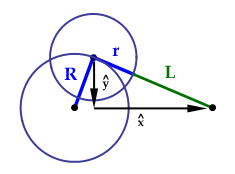Figure 1: The crank, hub, and the master rod and piston.

Although the master rod and hub are a single part, we want all of the rods to have the same length L. Because the rods are attached to the hub, we have to consider the hub's radius r. Therefore, the true length of the master rod when using the equations for a standard crank system is r + L. As we can see in figure 1, the vertical component of the master rod's vector is the $\widehat{\mathbf{y}}$ component of the crank vector, but it's facing the opposite direction:

$\widehat{\mathbf{y}}=-R \sin \theta$

Because we know the length of the master rod, r + L, we can apply the Pythagorean theorem and derive the $\widehat{\mathbf{x}}$ component:

$\widehat{\mathbf{x}}=\sqrt{\left(r+L\right)^2-\left(-R \sin \theta\right)^2}=\sqrt{\left(r+L\right)^2-\left(R \sin \theta\right)^2}$

Therefore, the vector for the master rod is given by:

$\overrightarrow{V_\text{rod}}=\sqrt{\left(r+L\right)^2-\left(R \sin \theta\right)^2}\ \widehat{\mathbf{x}} - R \sin \theta\ \widehat{\mathbf{y}}$

Generalizing the Rod Vectors

The position vector that defines the piston's path for the master rod is simply

$\overrightarrow{V_\text{crank}} + \overrightarrow{V_\text{rod}}$.

However, this doesn't help us formulate the paths for all of the pistons. In order to derive this equation, we have to consider the position vectors for the connection points that connect the rods to the hub. Although the master rod and hub are a single part, we will define them as being separate. This allows us to treat the master rod no different than the other rods, which is why we can derive a single equation that governs all of the piston paths.

Although we are redefining the equations for the rod vectors, we still need the vector equation for the master rod that we derived earlier. As the hub is rotated around the crank, the master rod points the hub towards the piston attached to it. This results in the hub being rotated by the angle defined by taking the arc tangent of the $\widehat{\mathbf{y}}$ component divided by the $\widehat{\mathbf{x}}$ component of the vector $\overrightarrow{V_\text{rod}}$.

$\rho\left(\theta\right)=\tan^{-1}\left(\frac{-R \sin \theta}{\sqrt{\left(r+L\right)^2-\left(R \sin \theta\right)^2}}\right)$

It is important to note that since we have constrained the hub's rotation, we can treat the master rod as having a magnitude L and connected to the hub in the same manner as the other rods. This is because the constraint on the angle of the hub along with the piston being constrained to the x axis will always result in the master rod being at the same angle regardless if the hub and master rod are a single part or separate parts.

Now that we have the angle, $\rho \left(\theta\right)$, that the hub rotates as it goes around the crank, we can derive the vector valued function that defines the positions for the connection points where the rods connect to the hub. Because the pistons are radially distributed around the center of the crank, we'll define the angle the piston paths are rotated as $\phi$ where the angle of the piston path for the master rod is $\phi=0$. The equation that defines the angles for all of the pistons is

$\phi=\frac{2 \pi}{n}\,i$

where n is the number of pistons and i is the zero-based index of the piston path we are calculating. This let's us define the vectors for the connection points in the hub's local coordinate system as

$\overrightarrow{V_\text{hub}}=r \cos \left(\rho(\theta)+\phi\right)\widehat{\mathbf{x}}+r \sin\left(\rho(\theta)+\phi\right)\widehat{\mathbf{y}}$

$\overrightarrow{V_\text{hub}}=r \cos \left(\tan^{-1}\left(\frac{-R \sin \theta}{\sqrt{\left(r+L\right)^2-\left(R \sin \theta\right)^2}}\right)+\phi\right)\widehat{\mathbf{x}}\,+\,r \sin\left(\tan^{-1}\left(\frac{-R \sin \theta}{\sqrt{\left(r+L\right)^2-\left(R \sin \theta\right)^2}}\right)+\phi\right)\widehat{\mathbf{y}}$

with the actual position vectors for these connection points defined by

$\overrightarrow{H_{\phi}}=\overrightarrow{V_{\text{crank}}} + \overrightarrow{V_{\text{hub}}}$

Unfortunately, the forum software will not let me post the LaTex for the expanded form of $\overrightarrow{H_{\phi}}$ because the image is too big. As we can see in figure 2, the connection points on the hub trace paths that are similar to ellipses, but are actually egg shaped. Furthermore, it becomes apparent that if we know the position vectors for the connection points on the hub and constrain the other end of the rods to vectors that define the piston paths, we can redefine the coordinate system for each piston path such that the x axis is defined by $\widehat{\mathbf{u}}$ and the y axis is defined by $-\widehat{\mathbf{v}}$. If we look at this relationship for the master rod, we see that $\widehat{\mathbf{u}}$ is a vector that is defined along the x axis and $\widehat{\mathbf{v}}$ is a vector that is defined along the y axis but in the opposite direction.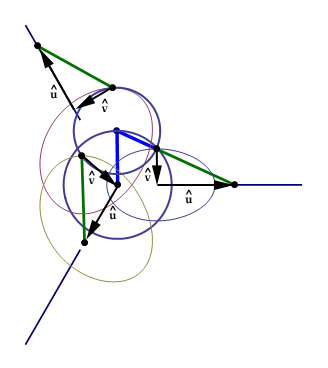Figure 2: The paths traced by the connection points on the hub.

Because the piston paths are radially distributed around the center of the crank, we can define the x axis for each path as

$\overrightarrow{\phi_{x}}=\cos \phi\ \widehat{\mathbf{x}}\ +\ \sin \phi\ \widehat{\mathbf{y}}$

This defines a vector that always point in the same direction as $\widehat{\mathbf{u}}$ for each path. The y axis must be perpendicular to the x axis.
So, the vector that defines the y axis for each path points in the opposite direction of $\widehat{\mathbf{v}}$. This allows us to define the y axis as

$\overrightarrow{\phi_{y}}=- \sin \phi\ \widehat{\mathbf{x}}\ +\ \cos \phi\ \widehat{\mathbf{y}}$

Now that we have defined vectors that represent coordinate axes for each piston path, we can project the position vectors for the connection points on the hub onto the vectors, $\overrightarrow{\phi_{x}}$ and $\overrightarrow{\phi_{y}}$, and derive the equation for the $\widehat{\mathbf{u}}$ and $\widehat{\mathbf{v}}$ component vectors. For a radial engine that has 3 pistons, the local coordinate systems for each piston path, where the path is now the x axis, is shown in figure 3.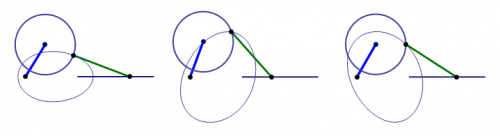Figure 3: The piston paths projected into their own coordinate system as defined by the vectors $\overrightarrow{\phi_{x}}$ and $\overrightarrow{\phi_{y}}$.

Because the magnitude of the vectors $\overrightarrow{\phi_{x}}$ and $\overrightarrow{\phi_{y}}$ are equal to one, the vector projection operation is simplified to a dot product:

$\text{proj}_{\ \overrightarrow{\phi_{x}}}\ \overrightarrow{H_{\phi}}=\frac{\overrightarrow{H_{\phi}}\cdot\overrightarrow{\phi_{x}}}{\overrightarrow{\phi_{x}}\cdot\overrightarrow{\phi_{x}}}\ \overrightarrow{\phi_{x}}=\left(\overrightarrow{H_{\phi}}\cdot\overrightarrow{\phi_{x}}\right)\ \overrightarrow{\phi_{x}}$

$\text{proj}_{\ \overrightarrow{\phi_{y}}}\ \overrightarrow{H_{\phi}}=\frac{\overrightarrow{H_{\phi}}\cdot\overrightarrow{\phi_{y}}}{\overrightarrow{\phi_{y}}\cdot\overrightarrow{\phi_{y}}}\ \overrightarrow{\phi_{y}}=\left(\overrightarrow{H_{\phi}}\cdot\overrightarrow{\phi_{y}}\right)\ \overrightarrow{\phi_{y}}$

Thus, the position vectors that define the connection points on the hub within the local coordinate system of the piston paths is

$\overrightarrow{J_{\phi}}=\left(\overrightarrow{H_{\phi}}\cdot\overrightarrow{\phi_{x}}\right)\ \overrightarrow{\phi_{x}}\ +\ \left(\overrightarrow{H_{\phi}}\cdot\overrightarrow{\phi_{y}}\right)\ \overrightarrow{\phi_{y}}$

where the length along the $\overrightarrow{\phi_{x}}$ axis is $\left(\overrightarrow{H_{\phi}}\cdot\overrightarrow{\phi_{x}}\right)$ and the length along the $\overrightarrow{\phi_{y}}$ axis is $\left(\overrightarrow{H_{\phi}}\cdot\overrightarrow{\phi_{y}}\right)$. An important thing to note is that although we transformed the piston paths along the axes defined by $\overrightarrow{\phi_{x}}$ and $\overrightarrow{\phi_{y}}$, the actual vectors $\overrightarrow{H_{\phi}}$, $\overrightarrow{\phi_{x}}$, and $\overrightarrow{\phi_{y}}$ are still in our original coordinate system for the radial engine. This allows all of our calculations in the coordinate systems of the piston paths to remain within our original coordinate system. Pretty cool huh?As can be seen in figure 3, we can now derive the values for the $\widehat{\mathbf{u}}$ and $\widehat{\mathbf{v}}$ components. Within the piston path's local coordinate system, the $\widehat{\mathbf{v}}$ component of the rod is defined by the $\widehat{\mathbf{y}}$ component of the position vector of the connection point on the hub except it is pointing in the opposite direction:

$\widehat{\mathbf{v}}=-\left(\overrightarrow{H_{\phi}}\cdot\overrightarrow{\phi_{y}}\right)$

Because the rod's length is L, we can apply the Pythagorean theorem like we did for the standard crank system and derive the value for the $\widehat{\mathbf{u}}$ component (because $\widehat{\mathbf{v}}$ is squared, we don't have to worry about the negative sign):

$\widehat{\mathbf{u}}=\sqrt{L^2-\left(\overrightarrow{H_{\phi}}\cdot\overrightarrow{\phi_{y}}\right)^2}$

This allows us to define the vector for the rods as

$\overrightarrow{V_{\text{rod}}}=\left(\sqrt{L^2-\left(\overrightarrow{H_{\phi}}\cdot\overrightarrow{\phi_{y}}\right)^2}\right)\ \overrightarrow{\phi_{x}}\ -\ \left(\overrightarrow{H_{\phi}}\cdot\overrightarrow{\phi_{y}}\right)\ \overrightarrow{\phi_{y}}$

Deriving the Solution to the Challenge

To define the piston path, all we have to do is add the $\overrightarrow{V_{\text{rod}}}$ vectors to the transformed vectors $\overrightarrow{J_{\phi}}$ for the connection points
on the hub:

$\overrightarrow{P_{\phi}}=\overrightarrow{J_{\phi}}+\overrightarrow{V_{\text{rod}}}$

Expand the vector equations:

$\overrightarrow{P_{\phi}}=\left(\overrightarrow{H_{\phi}}\cdot\overrightarrow{\phi_{x}}\right)\overrightarrow{\phi_{x}}\ +\ \left(\overrightarrow{H_{\phi}}\cdot\overrightarrow{\phi_{y}}\right)\overrightarrow{\phi_{y}}\ +\ \left(\sqrt{L^2-\left(\overrightarrow{H_{\phi}}\cdot\overrightarrow{\phi_{y}}\right)^2}\right)\ \overrightarrow{\phi_{x}}\ -\ \left(\overrightarrow{H_{\phi}}\cdot\overrightarrow{\phi_{y}}\right)\overrightarrow{\phi_{y}}$

The $\widehat{\mathbf{v}}$ components $\left(\overrightarrow{H_{\phi}}\cdot\overrightarrow{\phi_{y}}\right)\overrightarrow{\phi_{y}}$ and $-\left(\overrightarrow{H_{\phi}}\cdot\overrightarrow{\phi_{y}}\right)\overrightarrow{\phi_{y}}$ cancel out:

$\overrightarrow{P_{\phi}}=\left(\overrightarrow{H_{\phi}}\cdot\overrightarrow{\phi_{x}}\right)\overrightarrow{\phi_{x}}\ +\ \left(\sqrt{L^2-\left(\overrightarrow{H_{\phi}}\cdot\overrightarrow{\phi_{y}}\right)^2}\right)\ \overrightarrow{\phi_{x}}$

Simplify the result:

$\overrightarrow{P_{\phi}}=\left(\overrightarrow{H_{\phi}}\cdot\overrightarrow{\phi_{x}}\ +\ \sqrt{L^2-\left(\overrightarrow{H_{\phi}}\cdot\overrightarrow{\phi_{y}}\right)^2}\right)\ \overrightarrow{\phi_{x}}$

Now that we know the magnitude of the piston path's position vector along the $\overrightarrow{\phi_{x}}$ axis, we can transform the equation
back to the original coordinate system by expanding the $\overrightarrow{\phi_{x}}$ vector and arrive at the general solution to the challenge:

$\overrightarrow{P_{\phi}}=\left(\overrightarrow{H_{\phi}}\cdot\overrightarrow{\phi_{x}}\ +\ \sqrt{L^2-\left(\overrightarrow{H_{\phi}}\cdot\overrightarrow{\phi_{y}}\right)^2}\right)\ \left(\cos \phi\ \widehat{\mathbf{x}}\ +\ \sin \phi\ \widehat{\mathbf{y}}\right)$

After we expand the dot products and simplify the result, we get the solution to the challenge: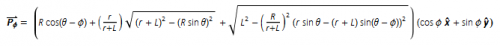As usual, I have attached the Mathematica 7 file for this challenge to this post. Because the bonus challenge is still in affect, I have removed the mathematics for its solution from the Mathematica fileEdited by Daedalus

##### Share on other sites

It's been over a month since I posted the solution to this challenge. I was hoping someone might try their hand at the bonus challenge given that it was much much easier than the challenge itself (especially considering you had the answer for the challenge). Anyways, as promised, here is the solution to the bonus challenge:

Defining The Mathematics for the Hub

This system is similar to a radial engine with a master rod except that all of the rods are individual components seperate from the hub.
Again, we are not going to consider forces exerted by the pistons according to their firing sequence. This would overcomplicate the
challenge. Instead, we will assume that the hub geometry is not rotated as it changes position around the crank. This is different than
an engine that contains a master rod where the master rod rotates the hub as the piston attached to it changes position.

To solve for the equation that defines this system, we simply set $\rho\left(\theta\right) = 0$ and rework the equations that we defined for the radial engine
with a master rod. This let's us define the vectors for the connection points in the hub's local coordinate system as

$\overrightarrow{V_\text{hub}}=r \cos \left(\rho(\theta)+\phi\right)\widehat{\mathbf{x}}+r \sin\left(\rho(\theta)+\phi\right)\widehat{\mathbf{y}}=r \cos \left(\phi\right)\widehat{\mathbf{x}}+r \sin\left(\phi\right)\widehat{\mathbf{y}}$

with the actual position vectors for these connection points defined by

$\overrightarrow{\text{H}_{\phi}}=\left(R\cos\theta + r\cos\phi\right)\widehat{\mathbf{x}}+\left(R\sin\theta + r\sin\phi\right)\widehat{\mathbf{y}}$

As we can see, this greatly simplifies the equations, and by using the general solution to the challenge

$\overrightarrow{P_{\phi}}=\left(\overrightarrow{H_{\phi}}\cdot\overrightarrow{\phi_{x}}\ +\ \sqrt{L^2-\left(\overrightarrow{H_{\phi}}\cdot\overrightarrow{\phi_{y}}\right)^2}\right)\ \left(\cos \phi\ \widehat{\mathbf{x}}\ +\ \sin \phi\ \widehat{\mathbf{y}}\right)$

we can use these new equations and derive the solution to the bonus challenge, which is

$\overrightarrow{P_{\phi}}=\left(r+R\cos\left(\theta-\phi\right)+\sqrt{L^2+R^2\left(\cos^2\left(\theta-\phi\right)-1\right)}\right)\left(\cos\phi\,\widehat{\mathbf{x}}+\sin\phi\,\widehat{\mathbf{y}}\right)$I have attached the updated Mathematica file that contains the equations for the bonus challenge## Create an account

Register a new account